# Analytic Functions

Also found in: Dictionary.

## Analytic Functions

functions that can be represented by power series. This class of functions is of exceptional significance for the following reasons. First, it is sufficiently broad, embracing most of the functions encountered in the basic problems of mathematics and its applications to natural science and technology. The class of analytic functions includes elementary functions—polynomials, rational functions, exponential and logarithmic functions, power functions, trigonometric and inverse trigonometric functions, hyperbolic and inverse hyperbolic functions, and algebraic functions—and special functions—elliptic, cylindrical, and others. Second, the class of analytic functions is closed with respect to the basic operations of arithmetic, algebra, and analysis; that is, the application of arithmetic operations to functions of this class, the solution of algebraic equations with analytic coefficients, and differentiation and integration all lead only to analytic functions. Finally, analytic functions possess the important property of uniqueness: every analytic function forms an “organically connected whole”; it is a “unique” function throughout its domain of existence. This property, which in the 18th century was considered inseparable from the very concept of a function itself, acquired fundamental significance after the establishment, in the first half of the 19th century, of the general view of a function as an arbitrary correspondence.

The theory of analytic functions was formulated in the 19th century, largely through the works of A. Cauchy, B. Riemann, and K. Weierstrass. A decisive role in the development of this theory was played by the “entry into the complex domain”—the transition from a real variable x to a complex variable z= x+ iy that can vary within an arbitrary region of the complex plane. The theory of analytic functions originated as the theory of functions of a complex variable; in a certain sense it is natural to consider the analytic functions and not arbitrary complex functions of two real variables x and y as functions of the complex variable z. The theory of analytic functions constitutes the basic content of the general theory of functions of a complex variable. For this reason the theory of analytic functions is frequently understood to mean the theory of functions of a complex variable.

There are different approaches to the concept of analytic-ity. Underlying the approach first developed by Cauchy and later greatly advanced by Riemann is the structural property of a function—the existence of a derivative with respect to a complex variable, or complex differentiability. This approach is closely allied to geometric considerations. A second approach, developed systematically by Weierstrass, is based on the possibility of representing functions by power series. It is thus associated with the analytical apparatus via which the function can be represented. The basic fact of the theory of analytic functions is the identity of corresponding classes of functions considered in an arbitrary domain of the complex plane.

Let us state some exact definitions. In what follows, z will always denote a complex number x + iy, where x and y are real numbers. Geometrically, the number z is represented as a point in the plane with coordinates x and y. A Euclidian plane whose points are identified with complex numbers is called a complex plane. Let D be a domain (open connected set) in the complex plane. If every point z of D is associated with a certain complex number w, then we say that a (single-valued) functions of the complex variable z is defined in D and we write w = f(z), z∊D. The function w = f(z) = f(x+iy) of the complex variable z∊D can be regarded as a complex function, defined in D, of two real variables x and y. Setting w = u + iv, where u and ν are real numbers, we note that the specification of such a function f is equivalent to specifying two real functions ϕ and ψ of the two real variables x and y defined in the same domain:

u = ɸ(x,y) v = ψ(x,y), (x,y)∊D

Let z be a fixed point in domain D. We give to z the arbitrary increment Δz = x + iΔy such that the point z+ Δz remains within D. Let us consider the corresponding increment in the function f: Δf(z) = f (z+ Δz) - f (z). If the difference quotient Δf (z)/Δ z has a limit as z→ 0—that is, there exists a complex number A such that for any >0 it will be true that ǀΔf(z)/Δz-A ǀ < ∊ whenever ǀΔzǀ < δ (δ = δ(∊)>0)—then the function f is said to be monogenic at point z, and the number A is its derivative at that point: A = f ‘(z) = df (z)/dz. A function that is monogenic at every point in D is said to be monogenic in domain D. If a function f is monogenic at a point z∊D, then f and the corresponding functions ϕ and ψ have partial derivatives with respect to x and y at this point. Here, ∂ f/∂x = ∂ϕ/∂x + ι(θψ/θx) and ∂f/∂y = dϕǀ∂y+ i’ψ/∂y). The derivative f ’(z)can be expressed in terms of the partial derivatives of f with respect to x and with respect to y; it is sufficient to calculate the limit of the ratio Δ/(z)/Δz in two different ways: as Δz = Δ x→ 0 and as Δz = iΔ y→ 0. Equating the corresponding expressions, we obtain ∂f/∂x = (1/i)∂f/∂y, or what is the same thing, ∂f/∂x + i(∂f/∂y) = 0. Going over to the ϕ and ψ, we can rewrite this equation as ∂ϕǀ∂x= θψ/ay and ∂ϕ/∂y = - ∂ψ/∂x. If f is monogenic in D,then the latter two relations are valid at every point of D; they are called the Cauchy-Riemann equations. It should be noted that these equations had already appeared in the 18th century in the works of D’Alembert and L. Euler in connection with the study of functions of a complex variable.

The monogeneity of the function f is equivalent to its differentiability in the sense of complex analysis. In this connection, by differentiability of/at a point z∊D we mean the possibility of representing the increment of/in the form Afiz) = Δz+α(Δz)Δz, where α(Δz) → 0 as Δz -> 0. The differential df(z)of the function/at the point z, which is equal to the principal part of Δz of its increment Afiz),is in this case proportional to dz =Δz and has the form f ’(z)dz.It is useful to compare the concepts of differentiability of a function f in real analysis and in complex analysis. In the former case, the differential df has the form (∂f/∂x)dx +(∂f/∂y)dy. It is convenient to rewrite this expression in complex form. To do so, we pass from the independent variables x, y to the variables z, z, which formally can be considered as new independent variables connected with the old ones by the relations z = x + iy and z = x - ry; thus viewed, the function/is sometimes written in the form (z̄.z).Expressing dx and dy in terms of dz and dz by the ordinary rules for calculating differentials, we arrive at df = (∂f/∂z) dz + (∂f/∂z) dz, where ∂f/∂z = (½) (∂f/∂x - ∂if/∂y) and ∂f/∂z = (½) (∂f/∂x + i∂f/∂y) are the (formal) derivatives of the function f with respect to z and z, respectively. From this it is not difficult to conclude that complex differentiability of a function/exists if and only if that function is differentiable in the sense of real analysis and the equation dfldz= 0 is valid; this equation is an abbreviated form of the Cauchy-Riemann equations. Here,

∂f/∂z = f’ = df/dz

The equation ∂f/∂z̄ = 0 indicates that those and only those functions f that are regarded formally as functions of independent variables z and z̄ (“depend only on z,” are “functions of the complex variable z”) are differentiable in the sense of complex analysis.

The integral of the function f = ϕ + iψ along the (oriented and rectifiable) curve Γ can be determined with the help of the line integral

rf (z) dz = ∫rf dx + if dy = ∫r ɸ dx − ψ dy + ir ψ dx + ɸ dy

A central position in the theory of monogenic functions (in Cauchy’s theory) is occupied by Cauchy’s integral theorem: if a function is monogenic in a simply connected domain D, then ∫rf (z) dz = 0 for any closed curve Γ lying in that domain. In an arbitrary domain D, the same assertion is valid for closed curves Γ which can be shrunk to a point by continuous deformation without crossing the boundary of D. On the basis of Cauchy’s integral theorem, it is not difficult to prove Cauchy’s integral formula: if a function/is monogenic in a domain D and Γ is a simple closed curve belonging to D together with its interior D,then for any point zDf,The orientation of the curve Γ is presumed to be positive with respect to Dr.

Let a function f be monogenic in a domain D.Take an arbitrary point z0 in D and denote by γ a circle whose center is at Z0 and whose radius is ρ > 0, such that the circle and its interior K:ǀz-z0ǀ<p belong to D.Then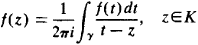Let us represent Cauchy’s kernal l/(r-z) for tey and z£K as the sum of an infinite geometric progression: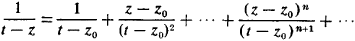Sincethe series converges uniformly with respect to t∊γ for any fixed z∊K. Integrating this series term by term—after multiplying by (1/2πi) f (t)—we get an expansion of f in a power seriesthat converges in the circle Kzz0ǀ<p.

Let us refine the concept of analyticity. Let/be a function defined in a domain D. This function is termed analytic (or holomorphic) at a point z0 of the domain if there exists a neighborhood of that point (a circle with center at z0) in which function/is representable as a power series:

f (z) = a0 + a1(zz0) + a2(z − z0)2 + . . . + an(z − z0)n + . . .

It was pointed out earlier that a function/ monogenic in D is also analytic in that domain. This does not hold true for an isolated point; for example, the function f (z) = ǀzǀ2 = zz̄ is monogenic at z0 = 0, but is nowhere analytic. On the other hand, a function f that is analytic at z0 in the domain D is monogenic at that point. Moreover, the sum of a convergent power series has derivatives of all orders (is infinitely differentiable) with respect to the complex variable z; the coefficients of the series can be expressed in terms of the derivatives of the function/at the point z0 by the formulas aR = f(n)(zo)/n! A power series written in the form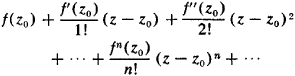is called the Taylor series of the function f at the point z0. Thus, the analyticity of a function f in a domain D means that at every point of D, the function f is infinitely differentiable and its Taylor series converges to it in a certain neighborhood of the point.

Consequently, the concepts of monogeneity and analyticity of a function in a domain are identical, and each of the following properties of a function f in a domain D can serve as a definition of the analyticity of f in that domain: monogeneity, differentiability in the sense of complex analysis, differentiability in the sense of real analysis, and fulfillment of the Cauchy-Riemann equations.

An important property of analytic functions is expressed by the following uniqueness theorem: two functions that are analytic in a domain D and coincident on some set having a limit point in D coincide throughout D—that is, they are identical. In particular, a nonidentically zero function that is analytic in the domain can only have isolated zeros.

If E is an arbitrary set (in the complex plane and, in particular, on the real line), then the function f(z), z∊E, is said to be analytic on the set E if every point of the set has a neighborhood at whose intersection with set E the function f is representable as a convergent power series. Actually, this means that f is analytic on a certain open set containing Ef more precisely, there exists an open set containing E and on that set there exists an analytic function/that coincides with f on E. For open sets, the concept of analyticity coincides with the concept of differentiability with respect to a set (monogeneity). This is not true, however, for the general case; in particular, there are functions on the real line which not only have derivatives but are infinitely differentiable at every point, which are not analytic at any point of the real line. For example,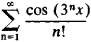On the other hand, an important property for confirming the uniqueness theorem of analytic functions is that of the connectivity of the set E. For this reason, analytic functions are usually considered in domains—that is, on open and connected sets.

An important role in the study of analytic functions is played by points at which the property of analyticity breaks down; these are the so-called “singular points” of an analytic function. We consider isolated singular points of (single-valued) analytic functions. Let/be an analytic function in a domain of type 0 < ǀzz0ǀ < p. In this domain, f is expanded in the Laurent series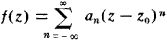which in the general case contains not only positive but also negative powers of zz0 - If in this expansion there are no terms with negative powers (an = 0 for n = −1, −2, . . .), then z0 is called an ordinary point of f. At an ordinary point, there exists a limit,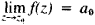, which is finite; setting f (z0) = a0, we obtain a function that is analytic throughout the circle ǀzz0ǀ < p. If the Laurent series of the function f contains only a finite number of terms with negative powers of zz0: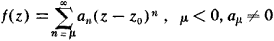then the point z0 is called a pole of the function f (of order μ); the pole z0 is characterized by the fact that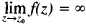.

If the Laurent series contains an infinite number of negative powers of zz0, then z0 is called an essential singular point; at such points, the function f has neither a finite nor an infinite limit. If z0 is an isolated singular point of a function f, then the coefficient a−1 in its Laurent expansion is called the residue off at z0.

Functions representable in the form of a ratio of two functions that are analytic in a domain D are said to be meromor-phic in D. A function that is meromorphic in a domain is also analytic in that domain, except possibly for a finite or de-numerable set of poles. The values of a meromorphic function are considered to be equal to infinity at the poles. If we admit such values, then functions that are meromorphic in a domain D can be defined as functions which in the neighborhood of every point z0 of D are representable by a power series in zz0 containing a finite (and dependent on z0) number of terms involving negative powers of zz0

Frequently, functions that are analytic (holomorphic) in a domain D and those that are meromorphic are both said to be analytic in D. In this case, holomorphic functions are also referred to as regular analytic functions or, simply, regular functions. The simplest class of analytic functions are those that are analytic throughout the plane. These are called integral (entire) functions. Integral functions are represented by series of the type

a0 + a1z + a2z2 + . . . . + anzn + . . . .

which converge throughout the complex plane. This group includes polynomials in z, the functions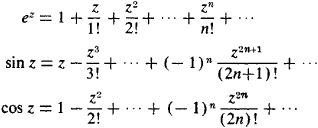Functions meromorphic throughout the plane—that is, representable in the form of ratios of integral functions—are called meromorphic functions. Such are the rational functions of z (ratios of polynomials), tan z = sin z/cos z, cot z = cos z/sin z, the elliptic functions, and others.

In the study of analytic functions, the associated geometric concepts are of great importance. The function w = f (z), zD, can be regarded as a mapping of the domain D into the plane of the variable w. If f is an analytic function, then the image f (D) of the domain D is itself a domain (principle of conservation of the domain). From the condition of complex differentiability of a function f at a point z0D, it follows that if f’(zo) ǂ 0, the corresponding mapping preserves the angles at zo, both in absolute value and sign: in other words, the mapping is conformal. Thus there is a close link between analyticity and the important geometric concept of conformal mapping. If f is analytic in D and if f (z’) ǂ f (z’) when z’ ǂ z” (such functions are called one-sheet functions), then f’(z0) ǂ 0 in D and f defines a one-to-one conformal mapping of D onto the domain G = f (D). The Riemann theorem—the basic theorem in the theory of conformal mapping—states that in any simply connected domain whose boundary contains more than one point, there exist one-sheet analytic functions that conformally map that domain onto a circle or half-plane.

Differentiating the Cauchy-Riemann equations, it is easy to see that the real and imaginary parts of the function f = ɸ + iψ, which is analytic in D, satisfy Laplace’s equation in that domain:that is, they are harmonic functions. Two harmonic functions connected by the Cauchy-Riemann equations are said to be conjugate. In a simply connected domain D, any harmonic function ψ has a conjugate function ψ and is thus the real part of a certain analytic function f in D. The relationships involving conformal mappings and harmonic functions underlie many applications of the theory of analytic functions.

All that has been said up to this point relates to single valued analytic functions/considered in a given domain D of the complex plane. Considering the possibility of extending a function/as an analytic function into a large domain leads to the concept of an analytic function regarded as a whole—in other words, throughout its natural domain of existence. With such an extension of a given function, its domain of analyticity may, in expanding, overlap itself and thus supply new values of the function at points in the plane where it has already been defined. Therefore, an analytic function regarded as a whole, generally speaking, proves to be mutiple-valued. Many of the problems encountered in the theory of functions (inversion of functions, finding an-tiderivatives, and construction of analytic functions with a given real part in multiply connected domains, solution of algebraic equations with analytic coefficients, and so on) necessitate the study of muliple-valued analytic functions, such asIn Z, arcsin Z, arctan Z, algebraic functions, and so forth. The regular process leading to a complete analytic function considered in its natural domain of existence was pointed out by Weierstrass; it goes by the name analytic continuation in the sense of Weierstrass.

A fundamental concept is that of a function element of an analytic function—a power series with nonzero radius of convergence. Such a function element W0,

a0 + a1(zz0) + a2(zz0)2 + . . . . + an(zz0)n + . . . .

defines a certain analytic function f in its circle of convergence K0. Let z1 be a point of K0, different from z0. Expanding f in a Taylor series with center at the point z1, we arrive at a new function element W1:

b0 + b1(zz1) + b2(zz1)2 + . . . . + bn(zz1)n + . . .

whose circle of convergence will be denoted by K1. In the area common to circles K0 and K1 the series. W1 converges to the same function as the series W0. If K1 extends beyond the bounds of the circle K0, then the series W1 defines a function specified by W0 on a certain set outside K0 (where the series W0 diverges). In this case, the function elements W1 is said to be a direct analytic continuation of the function element W0. Let W0, W1. . ., WN represent a chain of function elements such that Wi+1 is a direct analytic continuation of Wi(i = 1, . . . , N-1); then, WN is said to be an analytic continuation of the function element W0 (via the given chain of function elements). It may turn out that the center of circle KN belongs to circle K0, while WN is not a direct analytic continuation of the function element W0. In that case, the sums of the series WO and WN in the common area of circles K0 and KN will have different values; in this way analytic continuation can lead to new values of the function within the circle K0.

The aggregate of all function elements that can be obtained by a direct analytic continuation of the function element W0 constitutes a complete analytic function (in the sense of Weierstrass) generated by the function element W0. The union of their circles of convergence constitutes the (Weier-strassian) domain of existence of the function. From the theorem of uniqueness of an analytic function it follows that an analytic function in the Weierstrass sense is completely defined by specifying the function element W0. Any other function element belonging to this function may also be taken as the initial one, but this will not change the complete analytic function.

A complete analytic function f, regarded as a function of points in the plane that belong to its domain of existence D, is, generally speaking, multiple-valued. In order to eliminate this multivaluedness, the function/is regarded not as a function of points of the plane domain D, but as a function of points of a certain multisheeted surface R (lying above D) such that to every point of D there correspond as many points (projected on it) of the surface R as there are distinct values assumed by the function f at that point. On the surface R, f becomes a single-valued function. The idea of passing to such surfaces is one of the most remarkable and fruitful in all mathematics. It was originated by Riemann and the surfaces are called Riemann surfaces. Figures 1 and 2 are schematic illustrations of Riemann surfaces for the functions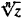and In z, respectively. The abstract definition of the concept of a Riemann surface made it possible to replace the theory of multiple-valued analytic functions with the theory of single-valued analytic functions on Riemann surfaces.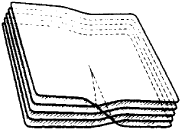Figure 1Figure 2

We fix a domain Δ belonging to the domain of existence D of a complete analytic function f; we also fix a function element W of f with center at a point of the domain Δ. The aggregate of all elements that can be obtained from an analytic continuation of the function element W via chains whose centers belong to Δ is called a branch of the analytic function f. A branch of a multiple-valued analytic function may turn out to be a single-valued analytic function in the domain Δ. Thus, for example, arbitrary branches of the functions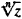and In z corresponding to any simply connected domain not containing the point O, are single-valued functions. At every point of the domain,has exactly n distinct branches and In z has infinitely many distinct branches. Isolating single-valued branches (by cuts in the domain of existence) and their study via the tools of the theory of single-valued analytic functions constitute one of the basic research techniques for investigating specific multiple-valued analytic functions.

The concept of an analytic function of several variables is introduced by means of multiple power series just as described above for analytic functions of a single variable. The properties of analytic functions of several complex variables are also analogous in many respects to those of analytic functions of a single complex variable. However, these functions exhibit a number of essentially new properties which have no counterparts in the theory of analytic functions of one variable. More general is the concept of analytic functions on complex manifolds; the concept of a complex manifold is a generalization of the Riemann surface to the multidimensional case.

### REFERENCES

Privalov, I. I. Vvedenie v teoriiu funktsii kompleksnogo peremen-nogo, 11th ed. Moscow, 1967.
Smirnov, V. I. Kurs vysshei matematiki, 8th ed., vol. 3, part 2. Moscow-Leningrad, 1969.
Markushevich, A. I. Teoriia analiticheskikh funktsii, 2nd ed., vols. 1–2. Moscow, 1967–68.
Lavrent’ev, M. A., and B. V. Shabat. Melody teorii funkstii kompleksnogo peremennogo, 3rd ed. Moscow, 1965.
Goluzin, G. M. Geometricheskaia teoriia funktsii kompleksnogo peremennogo, 2nd ed. Moscow, 1966.
Evgrafov, M. A. Analiticheskie funktsii, 2nd ed. Moscow, 1968.
Sveshnikov, A. G., and A. N. Tikhonov, Teoriia funktsii kompleks-noi peremennoi. Moscow, 1967.
Fuks, B. A. Teoriia analiticheskikh funktsii mnogikh kompleksnykh peremennykh, 2nd ed. Moscow, 1963.
Vladimirov, V. S. Metody teorii funktsii mnogikh kompleksnykh peremennykh. Moscow, 1964.
Markushevich, A. I. Ocherki po istorii teorii analiticheskikh funktsii. Moscow-Leningrad, 1951.
Matematika v SSSR za tridtsat’ let, 1917–1947. Moscow-Leningrad, 1948. Pages 319–14.
Matematika v SSSR za sorok let, 1917–1957, vol. 1. Moscow, 1959. Pages 381–510.

A. A. GONCHAR

References in periodicals archive ?
Removable singularities of analytic functions of several complex variables.
Janowski, "Some extremal problems for certain families of analytic functions.
Merkes, A coefficient inequality for certain classes of analytic functions, Proc.
Acharya, Univalence criteria for analytic functions and applications to hypergeometric functions, Ph.
For analytic boundaries and analytic function [gamma], the Nystrom method with the trapezoidal rule converges exponentially .
Keywords and Phrases: Analytic functions, Convex, Hadamard product, Subordination principle, Subordinating factor sequence, Univalent.
Abdulhalim, On a class of analytic functions involving the Salagean differential operator, Tamkang Journal of Mathematics, 23(1992), No.
lambda]z + (1 - [lambda]) [alpha] [member of] D), and let A (D) be the space of all single-valued and analytic functions in D that have a topology of uniform convergence on compact subsets.
Let H denote the class of analytic functions in the unit disc U = {z: [absolute value of z < 1} on the complex plane C.
Srivastava: Argument estimates of certain analytic functions defined by a class of multiplier transformations, Math.
The four lectures explain composition operators on the space of real analytic functions and Hardy-Orlicz spaces, shine a Hilbertian lamp on the bidisk, and consider several problems in perturbation theory.
Cross-platform analysis can be performed using Greenplum SQL and advanced analytic functions accessing data on HDFS.

Site: Follow: Share:
Open / Close